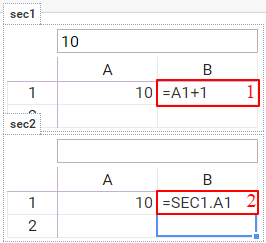# Cross section formulas

Formulas between sections have to use section name as a part of section address, see image below:1 internal formula, 2 cross section formula

Formula =A1+1 A1 refers to A1 cell in the same section, but formula =SEC1.A1 refers to cell A1 in section SEC1